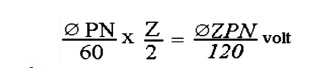Home | | Basic Electrical and Electronics Engineering | DC Generator E.M.F Equation

# DC Generator E.M.F Equation

Φ = flux/pole in weber Z = total number of armture conductors = No.of slots x No.of conductors/slot P = No.of generator poles A = No.of parallel paths in armature

DC GENERATOR E.M.F EQUATION

Let

Φ = flux/pole in weber

Z = total number of armture conductors = No.of slots x No.of conductors/slot

P = No.of generator poles

A = No.of parallel paths in armature

N = armature rotation in revolutions per minute (r.p.m) E = e.m.f induced in any parallel path in armature

Generated e.m.f Eg = e.m.f generated in any one of the parallel paths i.e E. Average e.m.f geneated /conductor = dΦ/dt volt (n=1)

Now, flux cut/conductor in one revolution dΦ = ΦP Wb No.of revolutions/second = N/60

Time for one revolution, dt = 60/N second

Hence, according to Faraday's Laws of Electroagnetic Induction,

E.M.F generated/conductor isFor a simplex wave-wound generator

No.of parallel paths = 2

No.of conductors (in series) in one path = Z/2

E.M.F. generated/path isFor a simplex lap-wound generator

No.of parallel paths = P

No.of conductors (in series) in one path = Z/P

E.M.F.generated/pathIn general generated e.m.fwhere A = 2 for simplex wave-winding A = P for simplex lap-winding

PRINCIPLE OF OPERATION

DC generator converts mechanical energy into electrical energy. when a conductor move in a magnetic field in such a way conductors cuts across a magnetic flux of lines and emf produces in a generator and it is defined by faradays law of electromagnetic induction emf causes current to flow if the conductor circuit is closed.

Study Material, Lecturing Notes, Assignment, Reference, Wiki description explanation, brief detail
Basic Electrical and electronics : Electrical Mechanics : DC Generator E.M.F Equation |